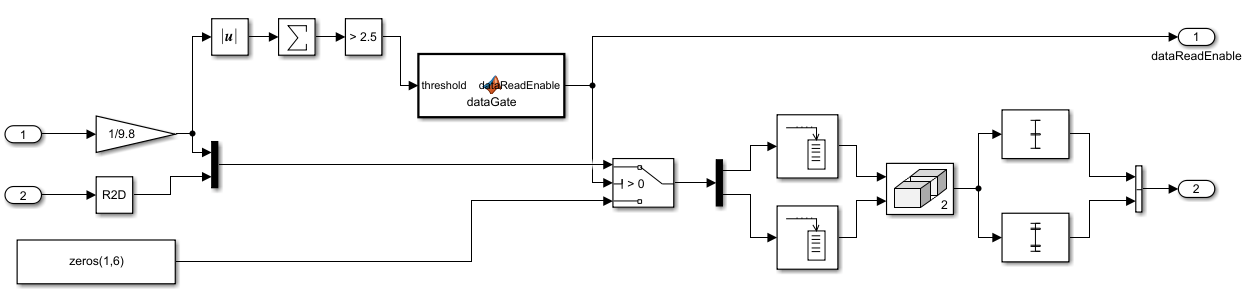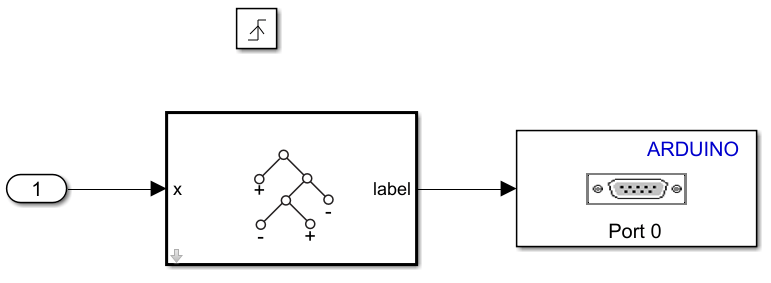# Identify Punch and Flex Hand Gestures Using Machine Learning Algorithm on Arduino Hardware

This example shows how to use the Simulink® Support Package for Arduino® Hardware to identify punch and flex hand gestures using a machine learning algorithm. The example is deployed on an Arduino Nano 33 IoT hardware board that uses an onboard LSM6DS3 6DOF IMU sensor to identify the hand gestures. The output from the machine learning algorithm, after identifying whether a hand gesture is a punch or a flex, is transmitted to the serial port where 0 represents a punch and 1 represents a flex.

### Required Hardware

• Arduino Nano 33 IoT board

• USB cable

### Hardware Setup

Connect the Arduino Nano 33 IoT board to the host computer using the USB cable.

### Prepare Data Set for Training Machine Learning Algorithm

This example uses a MATLAB code file named `capture_training_data` to measure the raw data for the flex and punch hand gestures. Open the file and configure these parameters.

1. Specify the acceleration threshold in the `accelerationThreshold` parameter. In this example, the threshold is set to `2.5`.

2. Create an `lsm6ds3` object and specify the number of samples read in a single execution of the read function. In this example, the parameter is set to `119`.

3. Specify the number of frames to be captured per gesture in the while loop. In this example, `100` frames are captured per gesture.

To capture the flex hand gestures, run this command in the MATLAB Command Window.

`flex = capture_training_data;`

Hold the Arduino hardware in the palm of your hand and throw a flex. To create a data set of 100 frames for a flex gesture, throw your hand for a flex 100 times. Observe the value of the `Gesture no.` being incremented every time you throw a flex in the MATLAB Command Window.

A 1-by-100 flex hand gesture data samples are available in Workspace that are read by the LSM6DS3 IMU sensor. Right-click flex in Workspace and save it as `flex_100.mat` in the same working directory of the example.

Follow the same procedure to create a data set of 100 frames for a punch gesture.

To capture the punch hand gestures, run this command in the MATLAB Command Window.

`punch = capture_training_data;`

Hold the Arduino hardware in the palm of your hand and throw a punch. To create a data set of 100 frames for a punch gesture, throw your hand for a punch 100 times. Observe the value of the `Gesture no.` being incremented every time you throw a punch in the MATLAB Command Window.

A 1-by-100 punch hand gesture data samples are available in Workspace that are read by the LSM6DS3 IMU sensor. Right-click punch in Workspace and save it as `punch_100.mat` in the same working directory of the example.

when you create your own data set for the flex and punch hand gestures, use the same names for the MAT-file in the `gr_script` MATLAB code file.

The `gr_script` MATLAB code file is used to preprocess and train the data set for flex and punch hand gestures, train the machine learning algorithm with the data set, and evaluate its performance to accurately predict these hand gestures.

To edit the `gr_script`.m file, run this command in the MATLAB® Command Window.

`edit gr_script;`

Use this MATLAB code file to prepare the Simulink model in the support package and then deploy the model on the Arduino hardware.

Alternatively, you can also load the flex and the punch data set from `gr_script` available in MATLAB.

```load flex_100 load punch_100```

To train and test the machine learning algorithm, 119 data samples are read from the accelerometer and the gyroscope. These 119 samples are grouped into 100 such frames, where each frame represents a hand gesture. Each frame has six values that are obtained from the X, Y, and Z axes of the accelerometer and the gyroscope, respectively. A total of 11,900 such observations are stored in the data set for two different hand gestures, flex and punch.

### Extract Features

Features are extracted by taking the mean and the standard deviation of each column in a frame. This results in a 100-by-12 matrix of observations for each gesture.

```f1 = cellfun(@mean,flex','UniformOutput',false); f2 = cellfun(@std,flex','UniformOutput',false); flexObs = cell2mat([f1,f2]); flexLabel = ones(100,1);```
```p1 = cellfun(@mean,punch','UniformOutput',false); p2 = cellfun(@std,punch','UniformOutput',false); punchObs = cell2mat([p1,p2]); punchLabel = 2*ones(100,1);```
```X = [flexObs;punchObs]; Y = [flexLabel;punchLabel];```

### Prepare Data

This example uses 90% of the observations to train a model that classifies two types of hand gestures and 10% of the observations to validate the trained model. Use cvpartition to specify a 10% holdout for the test data set.

```rng('default') % For reproducibility Partition = cvpartition(Y,'Holdout',0.10); trainingInds = training(Partition); % Indices for the training set XTrain = X(trainingInds,:); YTrain = Y(trainingInds); testInds = test(Partition); % Indices for the test set XTest = X(testInds,:); YTest = Y(testInds);```

### Train Decision Tree at Command Line

Train a classification model.

`treeMdl = fitctree(XTrain,YTrain);`

Perform a fivefold cross-validation for `classificationEnsemble` and compute the validation accuracy.

```partitionedModel = crossval(treeMdl,'KFold',5); validationAccuracy = 1-kfoldLoss(partitionedModel);```
`ans =`
`validationAccuracy = 1`

### Evaluate Performance on Test Data

Evaluate performance on the test data set.

`testAccuracy = 1-loss(treeMdl,XTest,YTest)`
`ans =`
`testAccuracy = 1`

The trained model accurately classifies 100% of the hand gestures on the test data set. This result confirms that the trained model does not overfit the training data set.

### Prepare Simulink Model and Calibrate Parameters

After you have prepared a classification model, use the `gr_script`.m file as a Simulink model initialization function.

To open the Simulink model, run this command in the MATLAB Command Window.

`open_system('arduino_machinelearning')`The Arduino Nano 33 IoT board has an onboard LSM6DS3 IMU sensor that measures linear acceleration and angular velocity along the X, Y, and Z axes. Configure these parameters in the Block Parameters dialog box of the LSM6DS3 IMU Sensor block:

1. Set the I2C address of the sensor to `0x6A` to communicate with the accelerometer and gyroscope peripherals of the sensor.

2. Select the Acceleration (m/s^2) and Angular velocity (rad/s) output ports.

3. Set the Sample time to `0.01`.

The 1-by-3 acceleration and angular velocity vector data is collected from the LSM6DS3 IMU sensor at the sample time you specify in the Block Parameters dialog box. This data is then preprocessed in the Preprocessing subsystem.

To open the subsystem, run this command in the MATLAB Command Window.

`open_system('arduino_machinelearning/Preprocessing')`The acceleration data is first converted from m/s^2 to g. The absolute values are then summed up and for every 119 data values that are greater than a threshold of 2.5g, the dataReadEnable parameter in the MATLAB Function block becomes logically true. This acts as a trigger to the Triggered subsystem in the Classification area.

The angular velocity data is converted from radians to degrees. The acceleration and angular velocity data is multiplexed and given as an input to the Switch. For a data value greater than 0, the buffer stores the valid 119 gesture values corresponding to a punch and a flex. For data values less than zero, which indicates that no hand gesture is detected, a series of (1,6) zeros are sent to the output to match the combined acceleration and angular velocity data.

Configure this parameter in the Block Parameters dialog box of the Buffer block.

1. Set the Output buffer size parameter to `119`.

Features are extracted by calculating the mean and the standard deviation values of each column in a frame that results in a 100-by-12 matrix of observations for each gesture. These extracted features are further passed as an input to the Triggered subsystem in the Classification area.

To open the subsystem, run this command in the MATLAB Command Window.

`open_system('arduino_machinelearning/Triggered Subsystem')`The Rate Transition block transfers data from the output of the Preprocesssing subsystem operating at one rate to the input of the Triggered subsystem operating at a different rate.

The ClassificationTree Predict block is a library block from the Statistics and Machine Learning Toolbox™ that classifies the gestures using the extracted features. This block uses the `treeMdl` machine learning model to identify punches and flexes. This block outputs the predicted class label. The output is either a 0 or 1 corresponding to a punch or a flex, respectively.

The Serial Transmit block parameters are configured to their default values.

### Deploy Simulink Model on Arduino Board

1. On the Hardware tab of the Simulink model, in the Mode section, select Run on board and then click Build, Deploy & Start.

2. For easy analysis of the hand gesture data being recognized by the machine learning algorithm, run the following script in the MATLAB Command Window and read the data on the Arduino serial port.

```device = serialport(<com_port>,9600); while(true) rxData = read(device,1,"double"); if rxData==0 disp('Punch'); elseif rxData==1 disp('Flex'); end end```

Replace the `port` parameter with the actual com port of the Arduino board. The gesture detected by the machine learning algorithm is displayed on the Arduino serial port at the baud rate of 9600 where 0 represents a punch and 1 represents a flex.

3. Hold the hardware in the palm of your hand and throw a punch or a flex. Observe the output in the MATLAB Command Window.